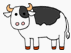Skill 3F
Understanding "How Many Are Left?"
A multi-step problem to determine if your student understands "how many are left" as well as filling in the operation in a subtraction number sentence.  This type of picture word problem is used by many textbooks as a method for teaching subtraction word problems. It is used as a way for preparing students for future word problems without picture clues. For example, this problem first shows the total animals and then next to that is a graphic of the number that are leaving.
This gives a better visual representation of the entire subtraction process and makes it easier for the student to associate the number sentence with the picture graphics.

There are 4 cows standing in the group.  If 2 cows walk away (as shown by the second group) - How many cows are left?

Before telling me the answer, tell me if we should add or subtract to solve the problem?  If you'd like I can ask the question again.
If your student understands "how many are left" by saying "subtract," then read the next part below the pictures, otherwise get the practice worksheet for this skill.

4 cows

2 walk awayIf we are going to subtract,
what sign should go in the box and what is the answer?

This shows if your student associates add and subtract with the proper symbols.2  =  ____